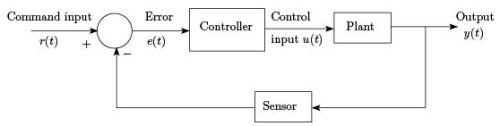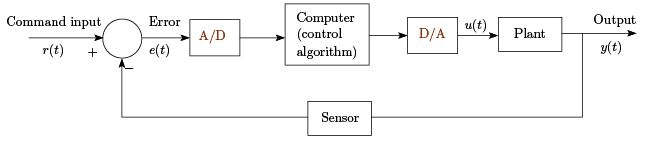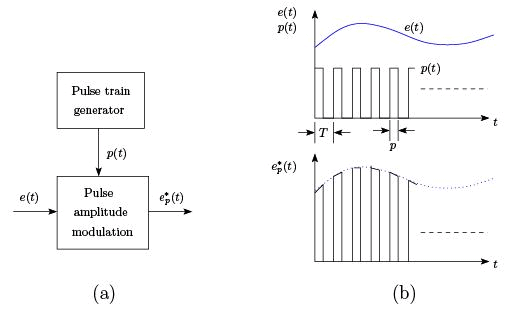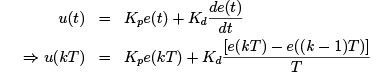Courses

# Lecture 1 - Introduction to Digital Control Electrical Engineering (EE) Notes | EduRev

## Electrical Engineering (EE) : Lecture 1 - Introduction to Digital Control Electrical Engineering (EE) Notes | EduRev

The document Lecture 1 - Introduction to Digital Control Electrical Engineering (EE) Notes | EduRev is a part of the Electrical Engineering (EE) Course Control Systems.
All you need of Electrical Engineering (EE) at this link: Electrical Engineering (EE)

Lecture 1 - Introduction to Digital Control, Control Systems

1 Digital Control System

A digital control system model can be viewed from diﬀerent perspectives including control algorithm, computer program, conversion between analog and digital domains, system performance etc. One of the most important aspects is the sampling process level.
In continuous time control systems, all the system variables are continuous signals. Whether the system is linear or nonlinear, all variables are continuously present and therefore known (available) at all times.A typical continuous time control system is shown in Figure 1.Figure 1: A typical closed loop continuous time control system

In a digital control system, the control algorithm is implemented in a digital computer. The error signal is discretized and fed to the computer by using an A/D (analog to digital) converter.
The controller output is again a discrete signal which is applied to the plant after using a D/A (digital to analog) converter. General block diagram of a digital control system is shown in Figure 2.
e(t) is sampled at intervals of T . In the context of control and communication, sampling is a process by which a continuous time signal is converted into a sequence of numbers at discrete time intervals. It is a fundamental property of digital control systems because of the discrete nature of operation of digital computer.
Figure 3 shows the structure and operation of a ﬁnite pulse width sampler, where (a) represents the basic block diagram and (b) illustrates the function of the same. T is the sampling period and p is the sample duration.Figure 2: General block diagram of a digital control systemFigure 3: Basic structure and operation of a ﬁnite pulse width sampler

In the early development, an analog system, not containing a digital device like computer,in which some of the signals were sampled was referred to as a sampled data system. With the advent of digital computer, the term discrete-time system denoted a system in which all its signals are in a digital coded form. Most practical systems today are of hybrid nature, i.e., contains both analog and digital components.
Before proceeding to any depth of the sub ject we should ﬁrst understand the reason behind going for a digital control system. Using computers to implement controllers has a number of advantages. Many of the diﬃculties involved in analog implementation can be avoided. Few of them are enumerated below.

1. Probability of accuracy or drift can be removed.

2. Easy to implement sophisticated algorithms.

3. Easy to include logic and nonlinear functions.

4. Reconﬁgurability of the controllers.

1.1 A Naive Approach to Digital Control

One may expect that a digital control system behaves like a continuous time system if the sampling period is suﬃciently small. This is true under reasonable assumptions. A crude way to obtain digital control algorithms is by writing the continuous time control law as a diﬀerential equation and approximating the derivatives by diﬀerences and integrations by summations.
This will work when the sampling period is very small. However various parameters, like overshoot, settling time will be slightly higher than those of the continuous time control.

Example: PD controller

A continuous time PD controller can be discretized as follows:where k represents the discrete time instants and T is the discrete time step or the sampling period. We will see later the control strategies with diﬀerent behaviors, for example deadbeat control, can be obtained with computer control which are not possible with a continuous time control.

1.2 Aliasing

Stable linear systems have property that the steady state response to sinusoidal excitations is sinusoidal with same frequency as that of the input. But digital control systems behave in a much more complicated way because sampling will create signals with new frequencies.
Aliasing is an eﬀect of the sampling that causes diﬀerent signals to become indistinguishable.
Due to aliasing, the signal reconstructed from samples may become diﬀerent than the original continuous signal. This can drastically deteriorate the performance if proper care is not taken.

2 Inherently Sampled Systems

Sampled data systems are natural descriptions for many phenomena. In some cases sampling occurs naturally due to the nature of measurement system whereas in some cases it occurs because information is transmitted in pulsed form. The theory of sampled data systems thus has many applications.

1. Radar: When a radar antenna rotates, information about range and direction is naturally obtained once per revolution of the antenna.

2. Economic Systems: Accounting procedures in economic systems are generally tied to the calendar. Information about important variables is accumulated only at certain times,
e.g., daily, weekly, monthly, quarterly or yearly even if the transactions occur at any point of time.

3. Biological Systems: Since the signal transmission in the nervous system occurs in pulsed form, biological systems are inherently sampled.
All these discussions indicate the need for a separate theory for sampled data control systems or digital control systems.

3 How Was Theory Developed ?

1. Sampling Theorem: Since all computer controlled systems operate at discrete times only, it is important to know the condition under which a signal can be retrieved from its values at discrete points. Nyquist explored the key issue and Shannon gave the complete solution which is known as Shannon’s sampling theorem. We will discuss Shannon’s sampling theorem in proceeding lectures.

2. Diﬀerence Equations and Numerical Analysis: The theory of sampled-data system is closely related to numerical analysis. Diﬀerence equations replaced the diﬀerential equations in continuous time theory. Derivatives and integrals are evaluated numerically by approximating them with diﬀerences and sums.

3. Transform Methods: Z-transform replaced the role of Laplace transform in continuous domain.

4. State Space Theory: In late 1950’s, a very important theory in control system was developed which is known as state space theory. The discrete time representation of state models are obtained by considering the systems only at sampling points.

Offer running on EduRev: Apply code STAYHOME200 to get INR 200 off on our premium plan EduRev Infinity!

47 docs|61 tests

,

,

,

,

,

,

,

,

,

,

,

,

,

,

,

,

,

,

,

,

,

;